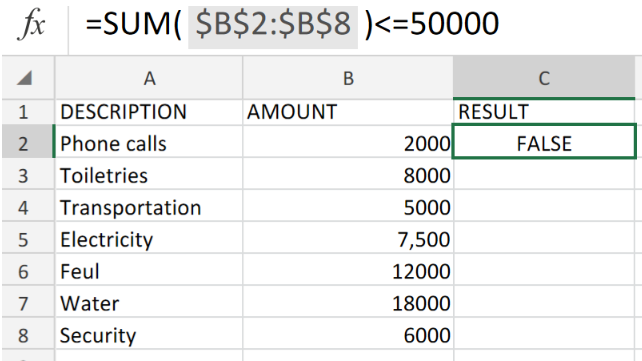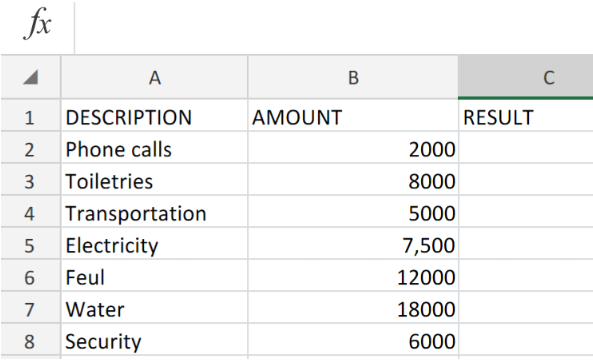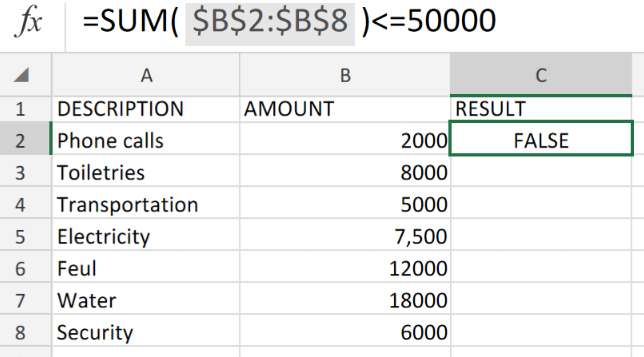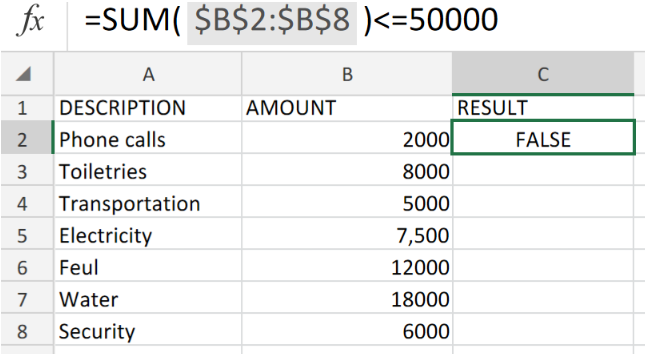Get instant live expert help with Excel or Google Sheets“My Excelchat expert helped me in less than 20 minutes, saving me what would have been 5 hours of work!”

#### Post your problem and you’ll get Expert help in seconds.

Your message must be at least 40 characters
Our professional Expert are available now. Your privacy is guaranteed.

# Excel DATA VALIDATION using SUM FunctionFigure 1. Excel DATA VALIDATION using SUM Function.

If we decide to restrict users to only input numeric values whose totally or sum does not exceed a specific amount within a selected range of cells, then we can achieve this by using a custom Data Validation formula.

## Formula Syntax

`=SUM(\$C\$2:\$C\$9)<=50000`

The Data Validation in Excel, requires us to set a custom formula based on the SUM function. This formula is to specify the amount users are limited to.

## How to use the SUM Function Data Validation in Excel.

1.In our worksheet example below, we have a list of a breakdown of monthly budgetary expenses for an office.Figure 2. Excel DATA VALIDATION using SUM Function.

Be sure to provide an empty cell for Excel to return the desired validation result.

1. Our purpose here is to limit users from entering a total monthly budgetary amount greater than or equal to \$50,000.

The custom Data Validation formula we will enter into cell C2 of our worksheet example, is as follows;

`=SUM(\$B\$2:\$B\$8)<=50000`Figure 3. Excel DATA VALIDATION using SUM Function.

Our validation result was returned as FALSE meaning, the total of the amounts within the cell range B2:B8 is greater than our specified limit of \$50,000.Figure 4. Final Result.

## Instant Connection to an Expert through our Excelchat Service:

Our live Excelchat Service is here for you. We have Excel Experts available 24/7 to answer any Excel questions you may have. Guaranteed connection within 30 seconds and a customized solution for you within 20 minutes.

### Did this post not answer your question? Get a solution from connecting with the expert.Another blog reader asked this question today on Excelchat:
Related blogs
Solution examplesI am trying to have a tab change color when the Sum of a row = 0. I am using code on the tab but it appears to be confused because the value of the given cell is not 0 it is "=SUM(G4:G17)"
Solved by S. F. in 41 minsI need a formula to pop at the upper left corner of a spreadsheet. If I enter the month "January," I want the column number sum of January =SUM(AB11:AB75) from another section on the same excel page to pop right below the "January" cell, and not display the formula expression, but see the \$100.
Solved by T. Q. in 40 minsCan't add (SUM) in imported numbers from bank account
Solved by F. C. in 40 minsI need a formula to combine D2 to D100 to add together a column of numbers, then take away the same amount on the same row when column E is filled. i.e. column D is a price of an item, so the formula must calculate the total, then when the item is sold an 'a' is marked next to the item in column E, the formula then must deduct this amount from the total
Solved by X. W. in 20 minsI would like to have a diagram in a new sheet, where the horizontal axis is the days, as they are in column DX. Each day shall show the sum of all unique leads of that day, and I would like to be able to check via a box of checkboxes, which facilities are shown, the facilities are in column BC.
Solved by I. A. in 45 mins## Subscribe to Excelchat.coAnother blog reader asked this question today on Excelchat: